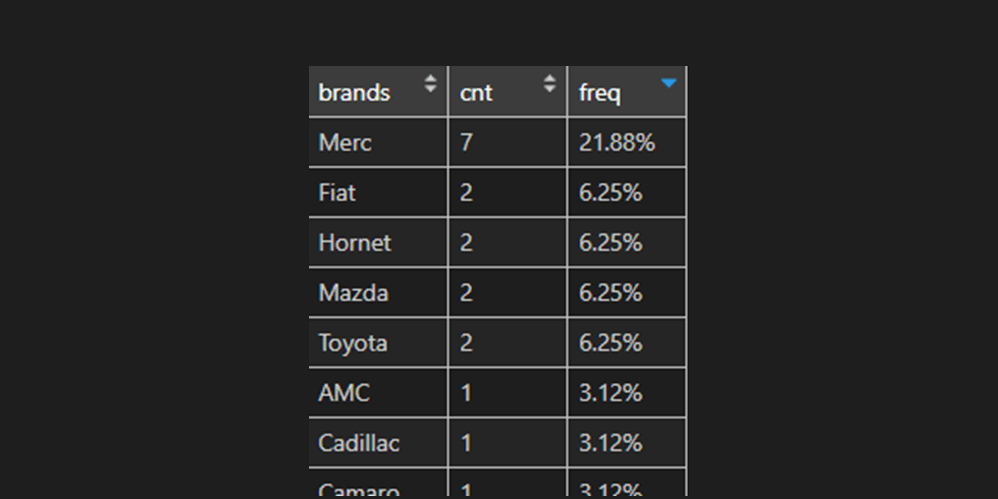# Calculate the percentage by a group in R, dplyr

Here is how to calculate the percentage by group or subgroup in R. If you like, you can add percentage formatting, then there is no problem, but take a quick look at this post to understand the result you might get.

## Build dataset

Here is a dataset that I created from the built-in R dataset mtcars. This process is useful to understand how to detect the first position of the space character in R and extract necessary information. In this case, car manufacturers and additional parameters of the cars.

```df <- data.frame("brands" = as.character(row.names(mtcars)), "cyl" = mtcars\$cyl)

df\$brands <-
ifelse(
is.na(stringi::stri_locate_first_fixed(df\$brands, " ")[,1]),
as.character(df\$brands),
substr(
df\$brands,
1,
stringi::stri_locate_first_fixed(df\$brands, " ")[,1] - 1
)
)

#  brands cyl
#1  Mazda   6
#2  Mazda   6
#3  Mazda   4
#4  Mazda   6
#5  Mazda   8
#6  Mazda   6```

## Calculate percentage within a group in R

Here is how to do the calculation by group using functions from package dplyr.

```require(dplyr)

g <- df %>%
group_by(brands) %>%
summarise(cnt = n()) %>%
mutate(freq = round(cnt / sum(cnt), 3)) %>%
arrange(desc(freq))

#  brands cnt  freq
#1   Merc   7 0.219
#2   Fiat   2 0.062
#3 Hornet   2 0.062
#4  Mazda   2 0.062
#5 Toyota   2 0.062
#6    AMC   1 0.031```

As you can see, the results are in decimal numbers, but if you want to get more visually appealing with percentage symbols, then here is how to do that. There is a good reason why I’m using the function from the formattable package.

```g <- df %>%
group_by(brands) %>%
summarise(cnt = n()) %>%
mutate(freq = formattable::percent(cnt / sum(cnt))) %>%
arrange(desc(freq))

#  brands cnt   freq
#1   Merc   7 21.88%
#2   Fiat   2  6.25%
#3 Hornet   2  6.25%
#4  Mazda   2  6.25%
#5 Toyota   2  6.25%
#6    AMC   1  3.12%```

## Calculate percentage within a subgroup in R

To calculate the percentage by subgroup, you should add a column to the group_by function from dplyr.

```g2 <- df %>%
group_by(brands, cyl) %>%
summarise(cnt = n()) %>%
mutate(freq = formattable::percent(cnt / sum(cnt)))```

If you’re interested in getting various calculations by a group in R, then here is another example of how to get minimum or maximum value by a group.

Categories R